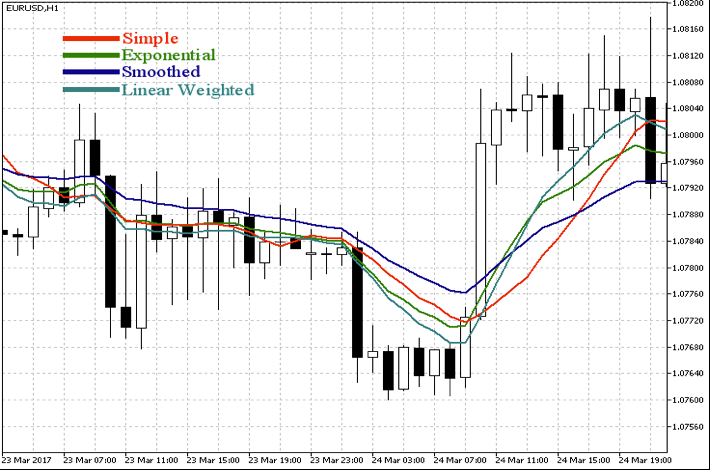#### Moving Average Probabilities

Moving Averages plays a simple but important role in the calculation of the Trend Intensity Indicator (TIR). Moving Averages are designed to provide a smoothing factor for a data set. I use an Exponential Moving Average (XMA) to provide a probability element to my TIR rating system. The XMA weights the calculation to the latest data and thus is more responsive to the latest data movement. This may or may not be what you consider appropriate, but this article simply presents the four basic types of Moving Averages and how I employ the type I use, the XMA.

There are various moving average calculations with the 4 main ones being simple, smoothed, weighted and exponential.

1. Simple Moving Average.Calculates an average of a number of prices. 20 periods divided by 20.
2. Smoothed Moving Average. This average gives the recent prices an equal weighting to the historic ones. The calculation does not refer to a fixed period, but rather takes all available data series into account.
1. Weighted Moving Average.The weighted moving average (WMA) gives you a weighted average of a number of prices, where the weighting decreases with each previous price. This works similarly to the XMA, but you calculate the WMA differently. This is done by multiplying each bar’s price by a weighting factor.
1. Exponential Moving Average.The exponential moving average (XMA) is a weighted average of a number of prices, where the weighting decreases exponentially with each previous price/period. In other words, the formula gives recent prices more weight than past prices.

They are all different and the decision is yours as to which you choose but what’s most important is understanding how the average you choose to use interacts with the price so you can understand its behaviour, define a methodology for using it, and benefit from its averaging attributes. The probability attribute tells us that if the average is rising and the price is above it this is a bullish sign and if below and falling is a bearish sign.My use of all the indicators that make up the TIR is to define simple rules that respect the purpose of providing a probability element to the Trend Intensity Indicator. In regard the moving average I employ only three rules. The readings are positive if the price is above the average and negative if below. My only variance is that a 1 week (based on weekly data) close above will give a lesser reading than 2 or more closes above and again the reverse is true on the downside. The purpose of this is to endorse a more purposeful break. Users may require more closes above or below the average depending on their own methodology. This is an attempt to wreak out the false breaks as often in a sideways market whipsaws can occur when using moving averages.

As this is only 1 element of 4 to my TIR its not taken on its own but rather in conjunction with other sentiment readings as an additional filter in discounting whipsaw behaviour in a sideways market.